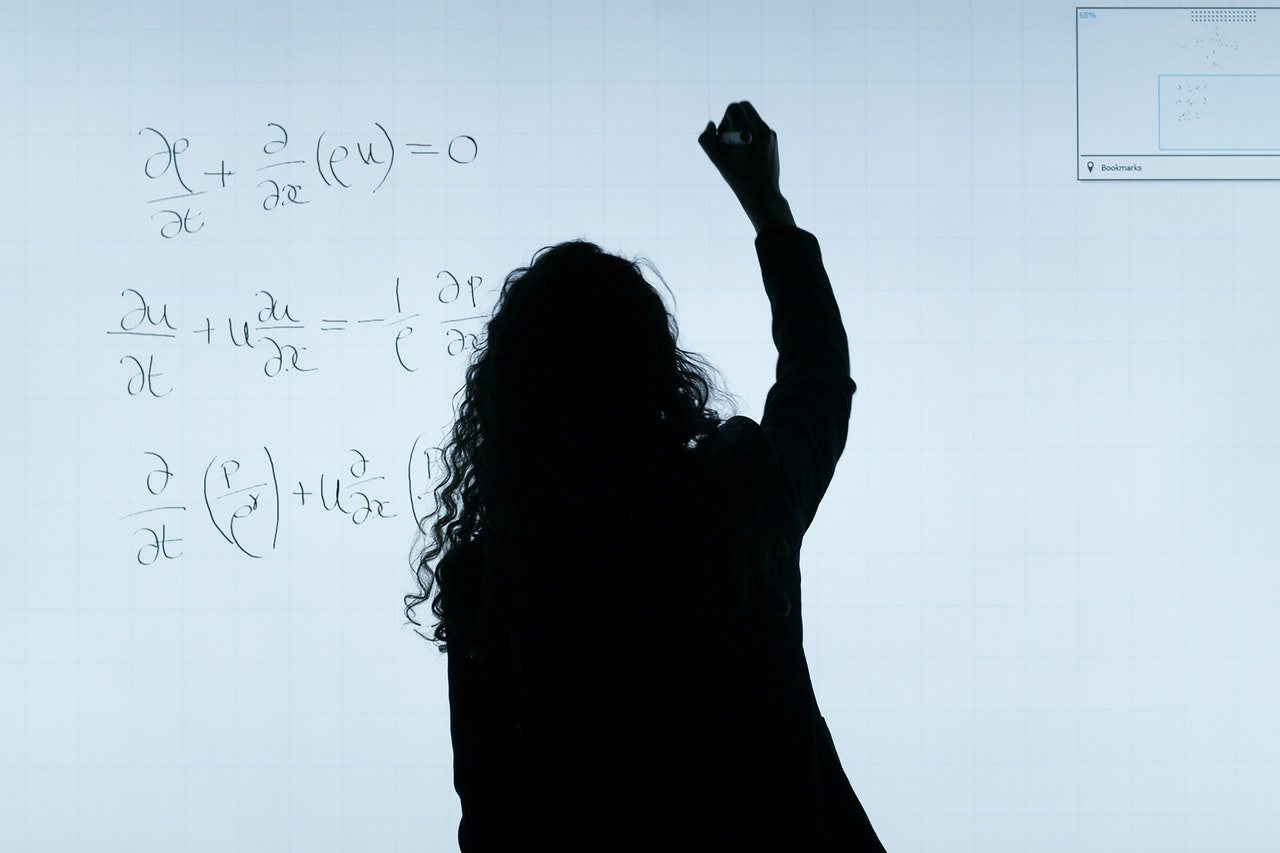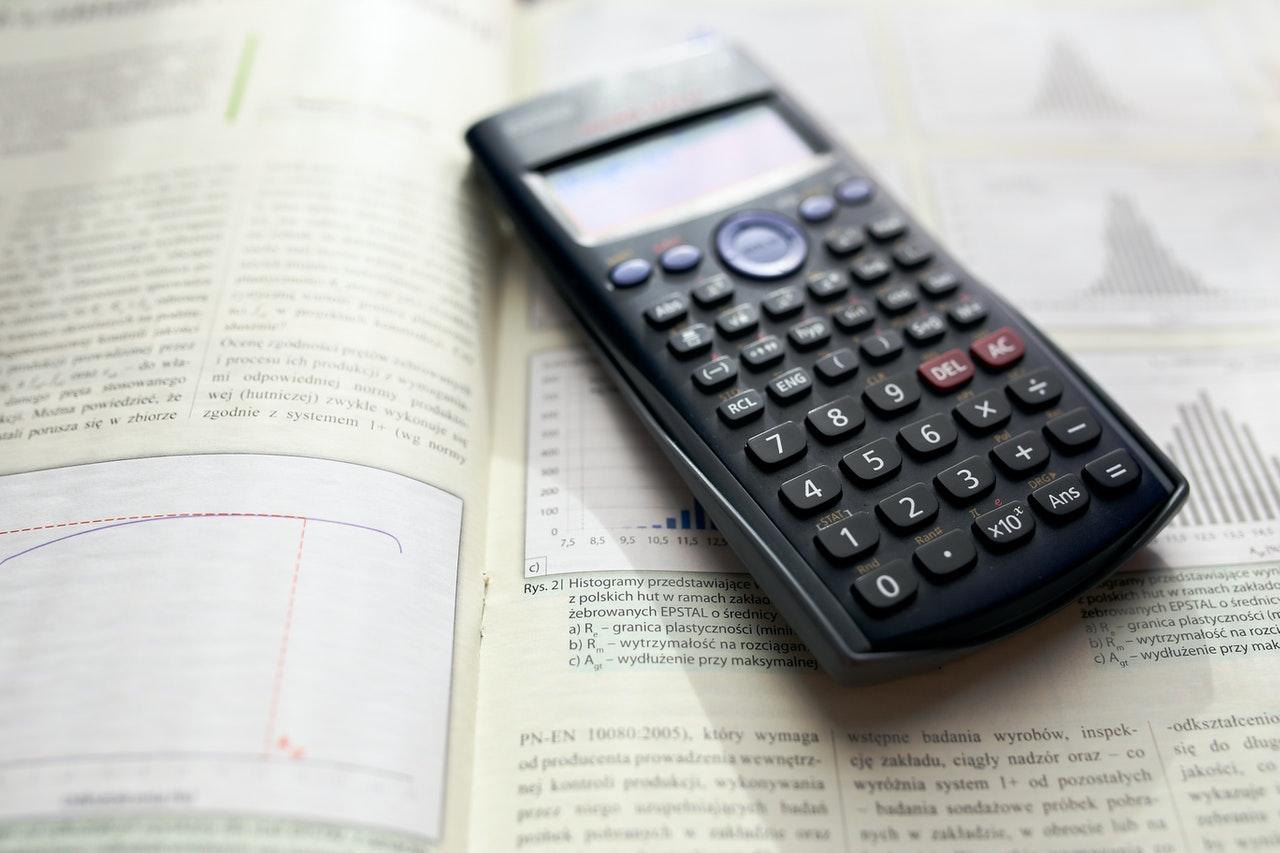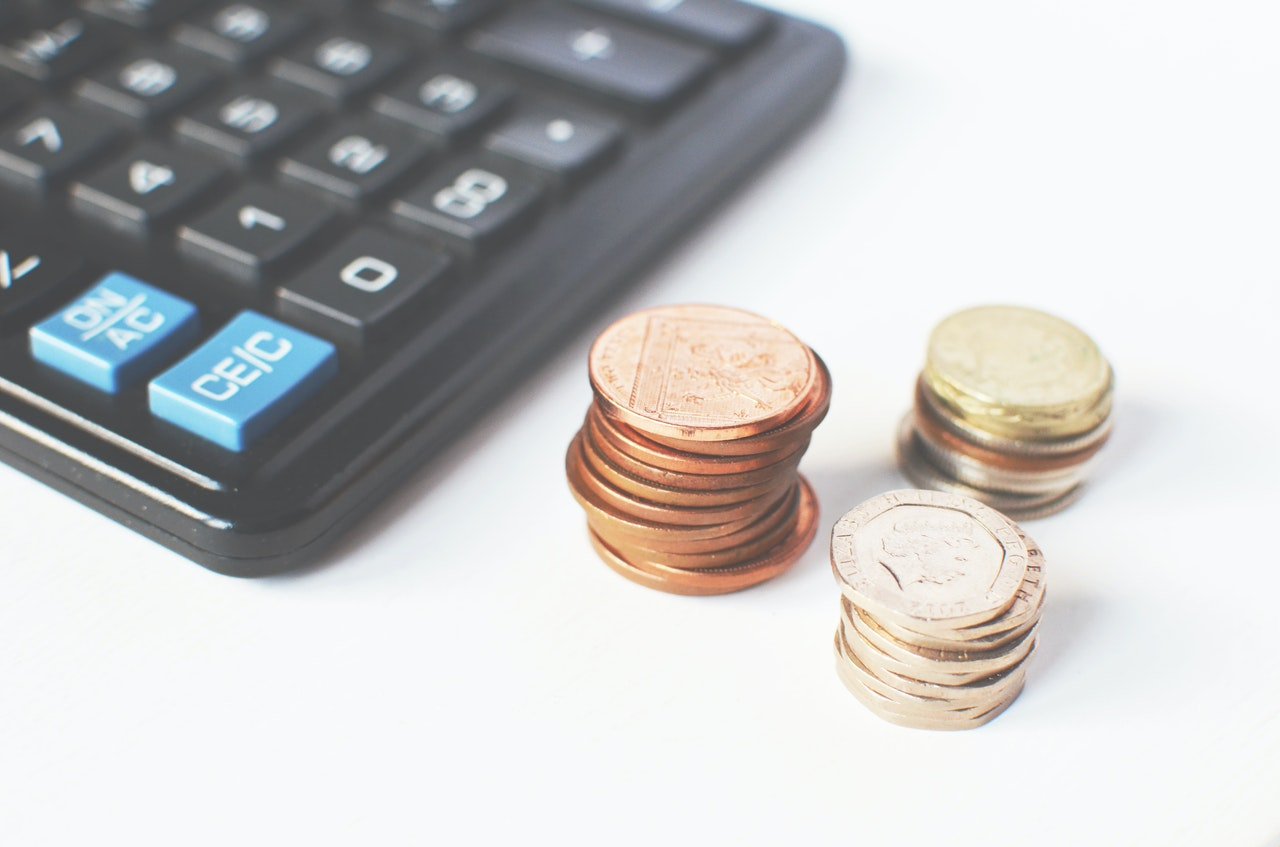• Home
• Enrolment

• FAQ

# Mathematics

### Mathematics is used to identify, describe and apply patterns and relationships. It provides a precise means of communication and is a powerful tool for solving problems both within and beyond Mathematics.

In addition to its practical applications, the study of Mathematics is a valuable pursuit, providing opportunities for originality, challenge and leisure. The aim of Mathematics in K–10 is to develop understanding and fluency through inquiry, explaining and connecting mathematical concepts. Students choose and apply problem-solving skills and mathematical techniques, communication and reasoning.#### Students will learn to

• Ask questions in relation to mathematical situations and their mathematical experiences;
• Develop, select and use a range of strategies, including the use of technology, to explore and solve problems;
• Develop and use appropriate language and representations to communicate mathematical ideas;
• Develop and use processes for exploring relationships, checking solutions and giving reasons to support their conclusions;
• Make connections with their existing knowledge and understanding using mathematics in the real world.

#### Areas studied

Number and Algebra

• Financial mathematics
• Rates and ratios
• Non-linear relationships
• Linear relationships
• Indices
• Algebraic techniques
• Equations
Statistics and Probability

• Data analysis
• Probability
Measurement and Geometry

• Surface area and volume
• Properties of geometrical figures
• Trigonometry

## Got a Question?

Fill out the form below and we will be in touch.